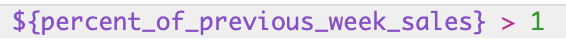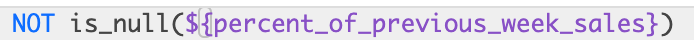# Using Table Calculations

Table calculations enable you to easily create on-the-fly metrics, which are similar to formulas found in spreadsheet tools like Excel. These columns will show up as green in the data table, rather than as blue (dimensions), or orange (measures).Table calculations can perform mathematical, logical (true/false), lexical (text-based), and date-based calculations on the dimensions, measures, and other table calculations in your query. The formulas that you use to execute these calculations are called Analytics Expressions.

# Table Calculations are Different From Regular Fields

Although table calculations are similar to the regular fields in Analytics, there are some important differences:

• Table calculations give anyone the ability to create new fields, as opposed to regular fields.
• Table calculations operate on the results from your query, as opposed to regular fields, which are part of the query itself. In other words, you’ll select a set of dimensions and measures and run your report as normal, then you can base table calculations on the data in that report.
• Although table calculations are easier to create than regular fields, they are not as easily controlled as regular fields. Since they can be created by anyone within your organization, they might not be the “official” calculations. Keep this tradeoff in mind as you decide between regular fields and table calculations, since one key advantage of Analytics is having a single source of truth!

# Using Table Calculations

## Creating Table Calculations in AnalyticsOn the Explore page, the dark Data bar has a Calculations button:This will bring up a Table Calculations pop-up where you can start constructing your custom metrics. The expression you create can evaluate to a number, date, string (text), or boolean (true/false).If you already have some table calculations defined, click the Add Table Calculation button to create another. You are able to add as many table calculations as you need.

Then, for each table calculation:

1. Rename your table calculation if desired.
2. Optionally, click Default Formatting to choose a predefined format or create a custom format for the results. If you create a custom format, use Excel-style formatting.
3. Start typing a Analytics Expression into a larger text box from your calculation. Analytics expressions can be quite simple, or they can use as many fields, functions and operators as your business logic requires. The next docs page, Creating Analytics Expressions, explains how to create Analytics Expressions and how the editor helps you.
4. If you are finished adding table calculations, click Save Table Calculations.

Your table calculation fields appear next to your dimensions and measures in the table. If you want to re-use your table calculations in the future be sure to save your Look, or copy the table calculation formula into another document.

## Sorting Table Calculation

To sort on a table calculation, click the field name at the top of the column, just as you would a dimension or measure.When Table Calculations cannot be Sorted

Sorting on a table calculation works similarly to sorting on a dimension or measure in Analytics. However, there are two important differences that prevent sorting in some scenarios:

• Table calculations are created after the data is retrieved from your database, which means that when you sort a table calculation, you can only sort the data that is already displayed.
• Some table calculations are applied to multiple rows within the same column (for example, when using an `offset()` function). In these cases, sorting the table calculation would change its results, and is therefore disabled.

The specific scenarios when you can’t sort a table calculation are:

1. Calculations that hit a row limit, as described below.
2. Sorting a dimension or measure after you’ve already sorted by a table calculation, as described below.
3. Sorting a table calculation that makes use of an offset, as described below.

### Calculations that Hit a Row Limit

If the number of rows in your query exceed the row limit that you’ve set, you will not be able to sort table calculations. This is because table calculations are only based on the rows that are displayed. Therefore, if you hit a row limit, the table calculation might be missing some rows that it should be sorting into your results. If you run into this issue, you can try increasing your row limit (up to 5,000 rows).

For example, the table below displays the ten top-selling brands in an ecommerce store, sorted by total sales. Notice that the 10 row limit has been reached, which you’re warned about by the yellow bar displayed at the top of the table:However, if we want to show the top ten brands by number of orders instead, you can see the results change (for example, TrendsBlue is new to the list):If you had tried to use table calculations to do this, they wouldn’t have searched through the undisplayed data, and would not have known that TrendsBlue existed.

### Sorting a Dimension or Measure After Sorting a Table Calculation

As indicated above, table calculations are only based on the rows that are displayed. In contrast, sorting by a dimension measure goes back to your database to make sure it finds the correct rows. As a result, you should start sorting with dimensions and measures. Then, when the correct data has been returned from your database, you can sort those results based on a table calculation.

### Calculations Using An Offset Function Cannot be Sorted

Any table calculation that makes use of an offset cannot be sorted, because the sort order of the rows would change the results of the offset.

For example, below is a table calculation that displays the percentage change in weekly sales for the Columbia brand:This of course only makes sense if the results are sorted by the week.

## Using Table Calculations in Visualizations

Just like regular dimensions and measures, table calculations are automatically displayed in visualizations.

In addition, you can use table calculations to decide which rows of your data should be displayed in a visualization. The example we’ll use to explore this feature is shown below, and includes weekly sales information about the brand Columbia. Note that the underlying data table includes the dimension Orders Created Week, the measure Order Items Total Sale Price, and a table calculation called Percent of Previous Week Sales that compares the revenue of each week against the previous week:We can now hide certain rows of data from showing up in the column chart. To do so, you’ll create a table calculation that evaluates to true or false, then hide the false values (which will appear as “no”s in your data table). You don’t want the formula to result in the word “true” or “false”, rather it should be a condition that is either true or false.

For example, suppose we only want to show weeks that had greater revenue than the previous week. We could create a table calculation called Percent of Previous Week Sales like this:

###Then we could create a table calculation called Exceeds Previous Week Sales like this:This will result in a table that looks like this:To hide all of the rows where a particular weekend did not exceed the revenue of the previous weekend, click the gear icon at the top left of the logical calculation and select Hide No’s from Visualization:The resulting visualization will now display only the weekends that exceeded the previous weekend:One common use case for this feature is hiding the first or last row from a visualization, since many types of analysis create bad rows at the beginning or end of a table. For example, when you are calculating running totals, have a partial day ending a date analysis, or are calculating a percent of the previous row like this example:

###To get rid of that row, simply create a new table calculation to filter out this null value:Then, hide the row as normal:# Common Problems and Pitfalls

• All the fields you use in your table calculations MUST be a part of your initial query.
• Formulas must be in lowercase. `ROUND` will not work, but `round` will.
• Table calculations will only operate over rows that are returned in your query. If there is a 500 row limit, the 501st row will not be considered.
• If you add a totals row to your data table, some table calculations that perform aggregations, such as calculations using `percentile` or `median`, might not add up as you expect. This is because table calculations calculate totals using the values in the total row, not using the values in the data column.
• Always use leading zeroes for decimals less than 1. For example, 0.95 will work, but .95 will cause an error.
• Using the Command-K or Ctrl+K shortcut will clear all table calculations, including custom fields. To reinstate your table calculations, hit the “back” button on your browser. You may also need to re-run your query.

# Conclusion

Table calculations provide a powerful way for any user of Analytics to manipulate and analyze their data, without approaching customer service. Next, you’re ready to go deeper into using Analytics Expressions in table calculations and custom filters.

Filtering and Limiting Data

Creating Analytics Expressions